# MultiPhase

Multi phase windings# Information

This information is part of the Modelica Standard Library maintained by the Modelica Association.

#### Symmetrical three phase system

Symmetrical three phases systems of currents (or voltages) consists of three sinusoidal sine waves with an angular displacement of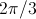.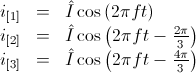,

Electrical three phase machines have (usually) symmetrical three phase windings which excite spatial magnetic potential with a spacial displacement of- with respect to the fundamental wave, see [Laughton02]. Such a symmetrical three phase system of currents (or voltages) can be represented by phasors, as depicted in Fig. 1(a). The associated three phase winding is depicted in Fig. 2(a). The winding axis are displaced by: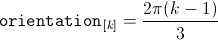So there is a strong coherence between angular displacement in the time and spatial domain which also applies to multi phase systems.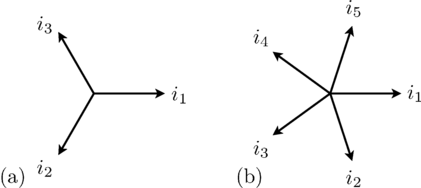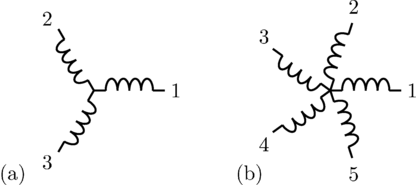#### Symmetrical multi phase system

In symmetrical multi phase systems odd and even phase numbers have to be distinguished.

##### Odd number of phases

For a symmetrical multi phase system with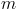phases the displacement in the time and spatial domain is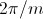, as depicted in Fig. 1 and 2.

Mathematically, this symmetry is expressed in terms of phase currents by: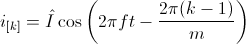The orientation of the winding axis of such winding is given by: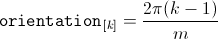##### Even number of phases

In the current implementation of the FundamentalWave library, phase numbers equal to the power of two are not supported. However, any other multi phase system with even an phase number,, can be recursively split into various symmetrical systems with odd phase numbers, as depicted in Fig. 3 and 4. The displacement between the two symmetrical systems is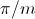. A function for calculating the symmetricOrientation is available.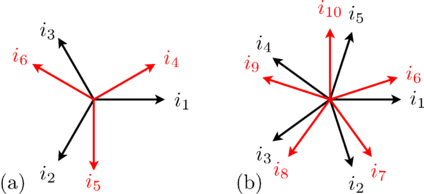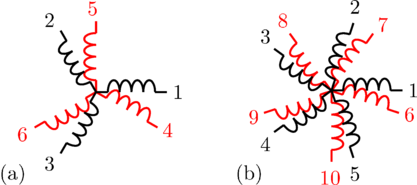#### Note

In a fully symmetrical machine, the orientation of the winding axes and the symmetrical currents (or voltages) phasors have different signs; see Fig. 1 and 2 for odd phase numbers and Fig. 3 and 4 for even phase numbers.